### Dice 1

1.  Observe the dots on the dice (one to six dots) in the following figures. How many dots are contained on the face opposite to the containing four dots?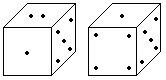A. 2 B. 3 C. 5 D. 6
2.  Two positions of a dice are shown below. When 3 points are at the bottom, how many points will be at the top?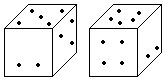A. 2 B. 5 C. 4 D. 6
3.  From the positions of a cube are shown below, Which letter will be on the face opposite to face with 'A'?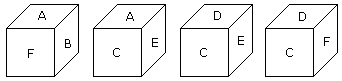A. D B. B C. C D. F
4.  Here 4 positions of a cube are shown. Which sign will be opposite to '+' ?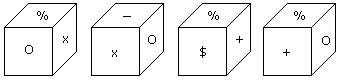A. % B. - C. x D. \$
5.  Two positions of a cubical block are shown. When 5 is at the top which number will be at bottom?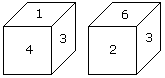A. 1 B. 2 C. 3 D. 4
6.  From the four positions of a dice given below, find the color which is opposite to yellow ?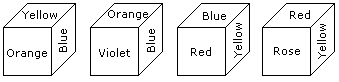A. Violet B. Red C. Rose D. Blue
7.  When the digit 5 is on the bottom then which number will be on its upper surface?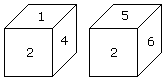A. 1 B. 3 C. 4 D. 6
8.  How many points will be on the face opposite to the face which contains 3 points?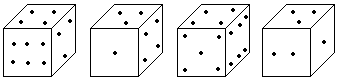A. 2 B. 4 C. 5 D. 6
9.  Which number is on the face opposite to 6?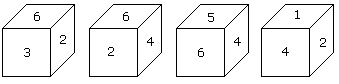A. 4 B. 1 C. 2 D. 3
10.  Two positions of a dice are shown below. When number '1' is on the top. What number will be at the bottom?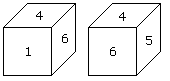A. 3 B. 5 C. 2 D. 6